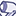# QlikView App Dev

Discussion Board for collaboration related to QlikView App Development.

Announcements
Join this live chat April 6, 10AM EST - QlikView to Qlik Sense REGISTER
cancel
Showing results for
Did you mean:Contributor II

What could be the possible problem when the if condition goes wrong?

I have this number 9590.101010...But Rank keep showing J.

Some numbers are correct, some are not.

Range1: 460

Range2: 13280

...

Range10: 134,509

If (value >= Range1 AND value < Range2) Then

Rank = "A"

ElseIf (value >= Range2 AND value < Range3) Then

Rank = "B"

ElseIf (value >= Range3 AND value < Range4) Then

Rank = "C"

ElseIf (value >= Range4 AND value < Range5) Then

Rank = "D"

ElseIf (value >= Range5 AND value < Range6) Then

Rank = "E"

ElseIf (value >= Range6 AND value < Range7) Then

Rank = "F"

ElseIf (value >= Range7 AND value < Range8) Then

Rank = "G"

ElseIf (value >= Range8 AND value < Range9) Then

Rank = "H"

ElseIf (value >= Range9 AND value < Range10) Then

Rank = "I"

Else  Rank = "J"

End If

1 Solution

Accepted SolutionsMVP & Luminary

It could be that either value or your various Range aren't interpreted as number or in the wrong way. Where did you try to apply this kind of logic because it don't look like valid qlik-code respectively how does your real code look like?

- Marcus

2 RepliesMVP & Luminary

It could be that either value or your various Range aren't interpreted as number or in the wrong way. Where did you try to apply this kind of logic because it don't look like valid qlik-code respectively how does your real code look like?

- MarcusContributor II
Author

Hi Marcus,

You're right. Tried to format all the Range and it works. Thanks